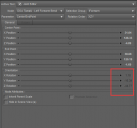# How to get joint orientation by daz script

I want get joint orientation by daz script, but fail.

Some joint result is true like Left Shoulder Bend,but some joint result is error.

I need help.(function () {

var pi = Math.PI;

return 180 / pi;

};

var bone = Scene.getPrimarySelection();

var nAngle = radialAngle();

var rot = bone.getLocalRot();

var rotOrder = bone.getRotationOrder();

var o = bone.getOrientation()

oEuler = o.getValue(rotOrder.firstAxis, rotOrder.secondAxis, rotOrder.thirdAxis);

oEulerD = oEuler.multiply(DzVec3(nAngle, nAngle, nAngle));

print(oEulerD)

})();

Post edited by Richard Haseltine on